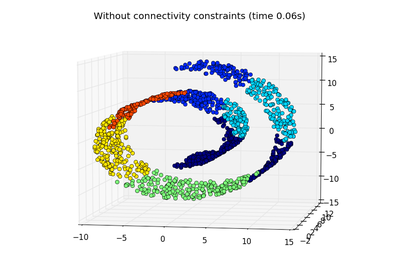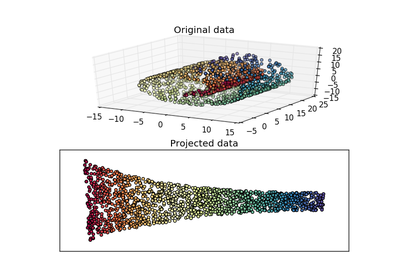# `sklearn.datasets`.make_swiss_roll¶

`sklearn.datasets.``make_swiss_roll`(n_samples=100, noise=0.0, random_state=None)[source]

Generate a swiss roll dataset.

Read more in the User Guide.

Parameters: n_samples : int, optional (default=100) The number of sample points on the S curve. noise : float, optional (default=0.0) The standard deviation of the gaussian noise. random_state : int, RandomState instance or None, optional (default=None) If int, random_state is the seed used by the random number generator; If RandomState instance, random_state is the random number generator; If None, the random number generator is the RandomState instance used by np.random. X : array of shape [n_samples, 3] The points. t : array of shape [n_samples] The univariate position of the sample according to the main dimension of the points in the manifold.

Notes

The algorithm is from Marsland .

References

 [R146] S. Marsland, “Machine Learning: An Algorithmic Perspective”, Chapter 10, 2009. http://seat.massey.ac.nz/personal/s.r.marsland/Code/10/lle.py

## Examples using `sklearn.datasets.make_swiss_roll`¶Hierarchical clustering: structured vs unstructured wardSwiss Roll reduction with LLE